Q.1. What are properties of a bar magnet ?

Ans ⇒ The following are the main properties of a bar magnet :

(i) Property of attraction : The magnet attracts small pieces of magnetic substances.

(ii) Directive properly or property of alignment : A freely suspended magnet always aligns itself along geographical N-S line.

(iii) Like poles repel and unlike poles always attract each other.

(iv) Property of pairs : Magnetic poles always exist in pairs and monopoles never exist.

(v) Repulsion is the surest test for distinguishing between a magnet and a piece of iron.

(vi) Property of induction : When a piece of ordinary magnetic material is brought near a bar magnet, it acquires the property of a magnet without any actual contact.

Q.2. Define the following :
(a) Hysteresis
(b) Residual magnetism or remanence or retentivity
(c) Coercivity
(d) Hysteresis loss

Ans ⇒ (a) It is defined as the lag of intensity of magnetisation behind the magnetising field during the process of magnetisation and diamagnetisation of a ferromagnetic material. It is shown in the figure. The curve ABCDEFA is known as hysteresis loop.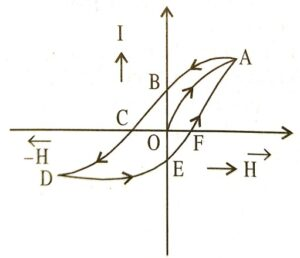(b) Retentivity : It is defined as the value of intensity of magnetisation of a material when the magnetising field is reduced to zero. e.g., OB represents retentivity of the material.

(c) Coercivity : It is defined as the value of the reverse magnetising field required to reduce the residual magnetism to zero.
eg., OC represents coercivity of material.

(d) Hysteresis loss : It is defined as the energy loss per unit volume per cycle of magnetisation. It is equal to the area under I – H curve.

Q.3. Give any Four properties of diamagnetic substances.

Ans ⇒ (i) They are weakly repelled by a magnet.
(ii) They are weakly magnetised in a direction opposite of that of applied magnetic field.
(iii) Magnetic flux density in them is less than in vacuum.
(iv) Magnetic susceptibility in their case has small negative value.
(v) Value of relative permeability in just less than 1.
(vi) They do not obey Curie’s law.

Q.4. State and illustrate Curie law in magnetism.

Ans ⇒ Curie law states that susceptibility of a material varies inversely with Curie discovered experimentally that intensity of magnetisation I off proportional to magnetic field B and inversely proportional to the the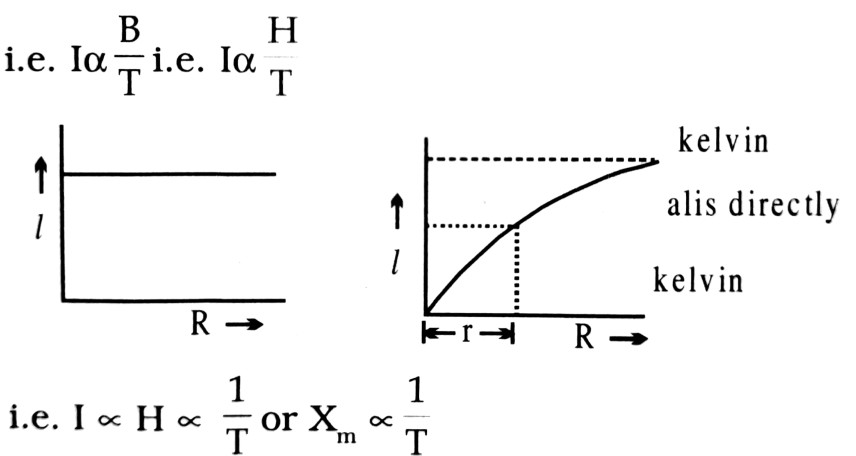Where Xm is called susceptibility of the material.
∴   Xm = C/T where C is called as Curie constant.

Q.5. What is tangent law in magnetism ?

Ans ⇒ Consider a magnet of pole strength m and magnetic length 21. This magnet is suspended or pivoted at its centre so that it can rotate freely about the point O. If this magnet is acted upon by two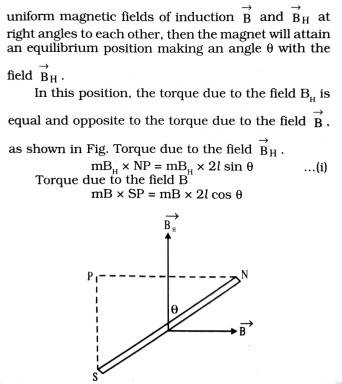In equilibrium position
mBH x 2l sin θ = mB x 2l cos θ
or,  B = BHtan θ
This relation is known as tangent law.

Q.6. Obtain an expression for the magnetic potential at a point due to an isolated magnetic pole.

Ans ⇒ Consider a very long magnet of pole strength m so that the effect of its S-pole at a point P be negligible, compared to that of the N-pole (Fig).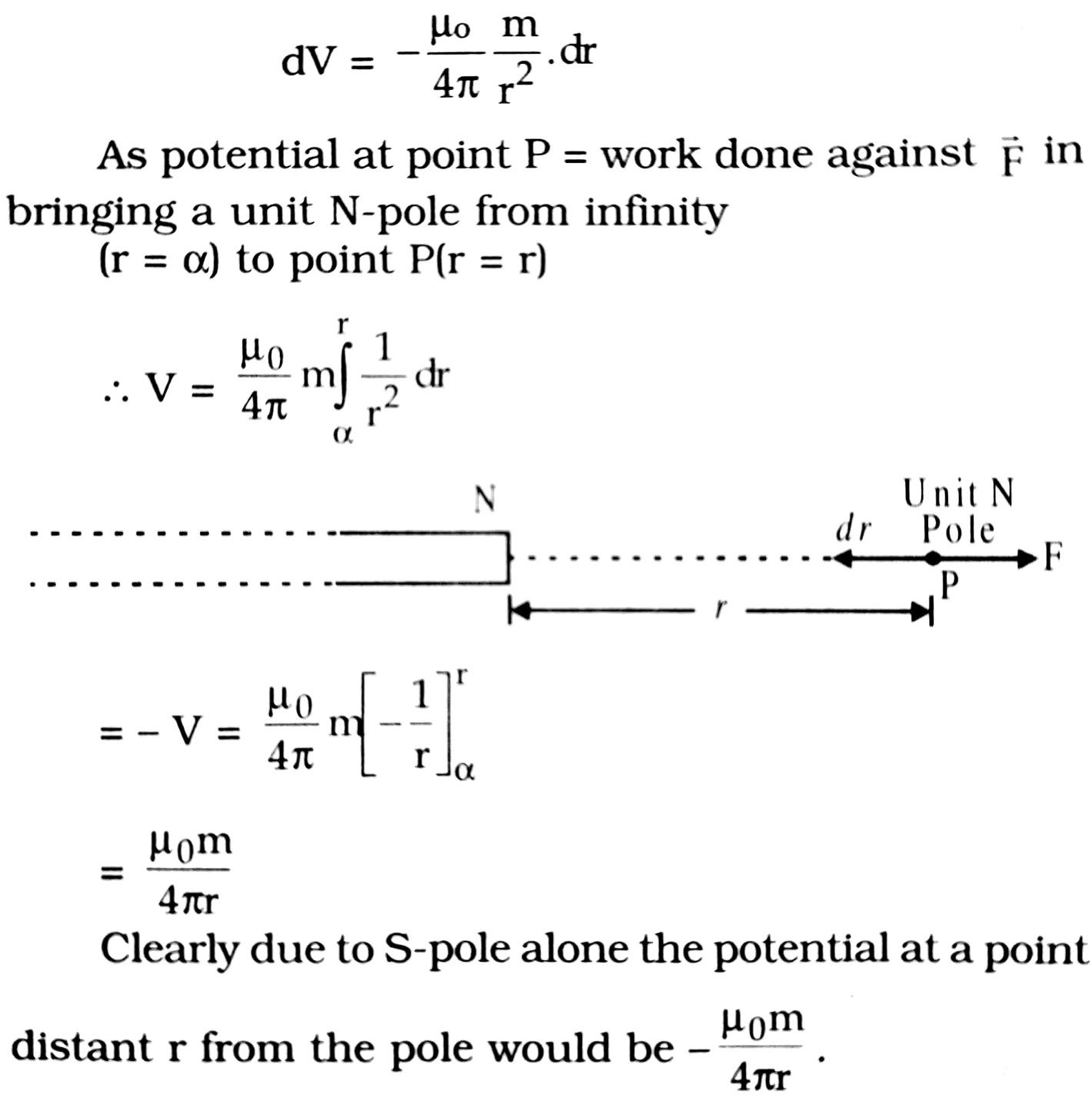Q.7. Give the differences between the magnetic properties of soft iron and steel.

Ans ⇒ The differences between the magnetic properties of soft iron and steel are as follow :

 S.N. Magnetic properties of soft iron Magnetic properties of steel 1 The soft iron gets strongly magnetic when magnetised by induction. Steel is feebly magnetic when magnetised by induction. 2 Soft iron can be magnetised by magnetic field. Steel requires strong magnetic field to get magnetised. 3 Soft iron can be demagnetised easily. Steel cannot be demagnetised easily. 4 Loss of energy during magnetisation and demagnetising a soft iron is very small. Loss of energy during magnetising and demagnetising steel is very high.

Q.8. Why is soft iron preferred in making an electromagnet ?

Ans ⇒ Soft iron has high value of retentivity and low value coercivity due to which it has less area of hysteresis loop, hence the loss in energy during magnetisation and demagnetisation is very small, that is why soft iron is used to make an electromagnet.

Q.9. State and illustrate Curie law in magnetism.

Ans ⇒ Curie law in magnetism. The law states : intensity of magnetisation I, of a magnetic material is
(i) directly proportional to magnetic induction (B).
and
(ii) inversely proportional to the temperature (T) of the material.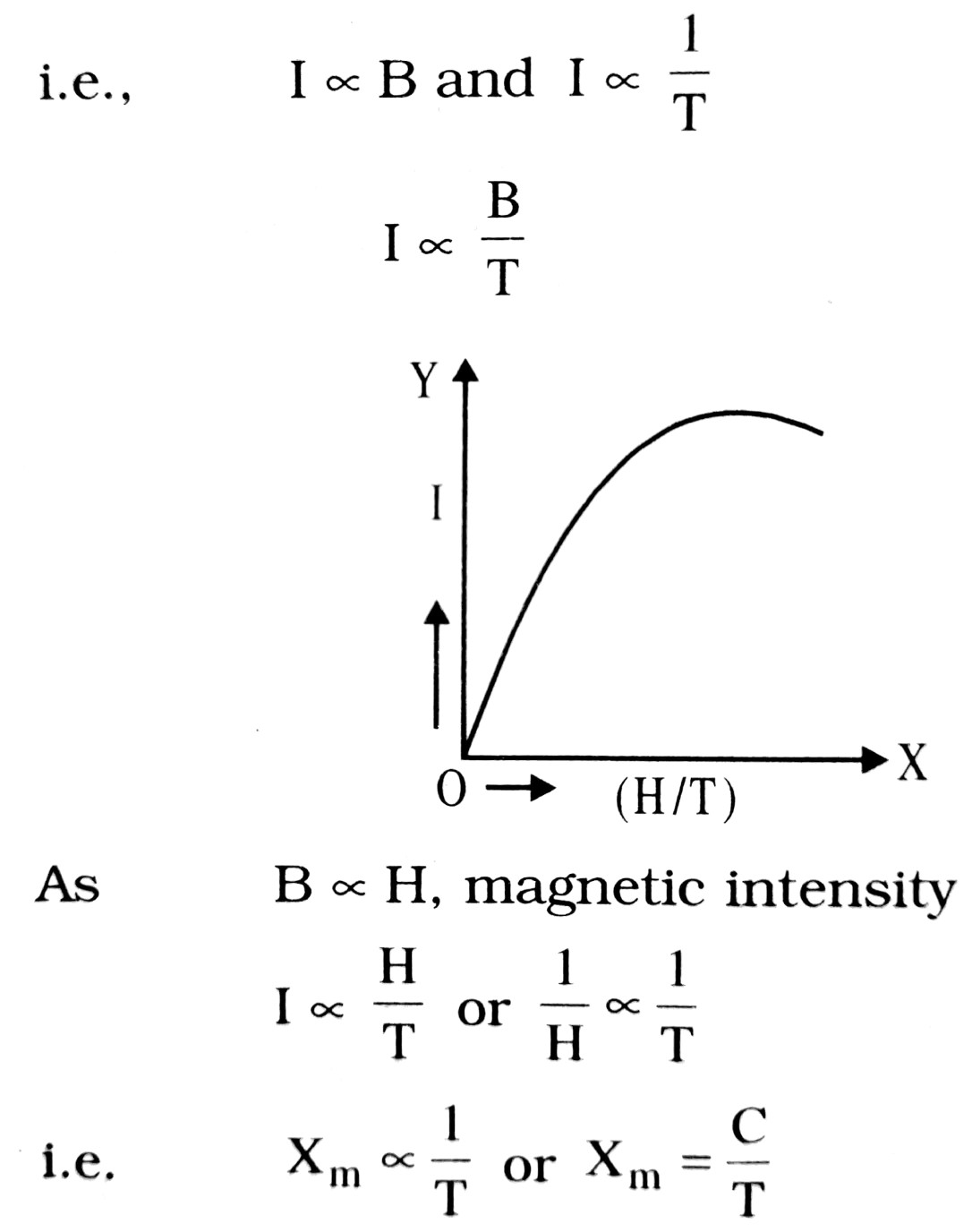…..(i)

where C is a constant of proportionality and is called Curie constant.
The law is physically reasonable as increasing H tends to align the elementary dipoles in the specimen increasing I, whereas increasing T,tends to interfere with this alignment, decreasing I.
The variation of I with (H/T) is shown in Fig. Curie’s law is well verified experimentally provided the ratio H/T is not too large. Saturation is reached when H/T reaches a certain limit, as is from the graph.

Q.10. How will a dia, para and a ferromagnetic material behave when kept in a non-uniform external magnetic field ? Give one example of each of these materials.

Ans ⇒ In a non-uniform magnetic field, a diamagnetic substance tends to move slowly from stronger to weaker parts of the field, a paramagnetic substance tends to move slow from weaker to stronger parts of the parts, while a ferromagnetic substance moves easily from weaker to stronger parts of the field.

Diamagnetic material : Bismuth.
Para magnetic material : Aluminium.
Ferro-magnetic material : Iron.

Q.11. Why is the plane of the coil of tangent galvanometer set in earth’s magnetic meridian ?

Ans ⇒ The tangent galvanometer is based on tangent law which requires two uniform magnetic
Fields perpendicular to each other. By keeping the i in the magnetic meridian, the magnetic field produced by the current carrying coil becomes perpendicular to the horizontal component (H) of in’s magnetic field. It is because magnetic field produced by a current carrying coll is perpendicular to the plane of the coil.

Q.12. If a compass needle be placed on the agnetic north pole of the earth, then how will it behave ?

Ans ⇒ At the magnetic north (or south) pole of the earth, the angle of dip is 90°. Therefore, only vertical component of earth’s magnetic field acts; the horizontal component of earth’s magnetic field being zero there. Consequently, the compass needle may stay in any direction.

Q.13. Define the terms magnetic inclination and horizontal component of earth’s magnetic field at a place. Derive the relationship between the two with the help of a diagram.

Ans ⇒ Inclination : The angle between the direction of the earth’s magnetic field at a place and the horizontal is called the angle of inclination at that place.

Horizonal componenent (H) : The total intensity of the earth’s magnetic field at any point m may be resolved into two rectangular components, one along the horizontal and the other along the vertical direction.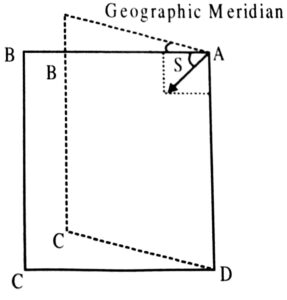The component of the resultant intensity of the earth’s magnetic field in the horizontal direction in magnetic meridian is called its horizontal component.
It is denoted by H. In Fig., the resultant intesity, i.e., R along AP has been resolved into rectangular components. Clearly the horizontal component,
H = R = cos δ               …(i)
and the vertical component
V = R = sin δ                …(ii)
Dividing (ii) by (i)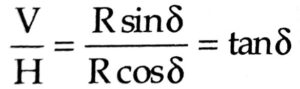Squaring (i) and (ii) and adding, we get
H2+V2=R2cos2δ+R2sin2δ
= R2(cos2 δ + sin2 δ) = R2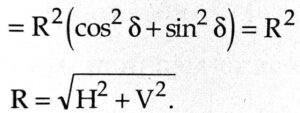Q.14. Why is diamagnetism independent of temperature ?

Ans ⇒ The induced magnetic dipole moment in a diamagnetic substance is always opposite to the applied magnetising field irrespective of the state of thermal agitation of the atoms. Therefore, diamagnetic effect does not depend upon temperature.

Q. 15. Explain differences between electric lines of force and magnetic lines of force.

Ans ⇒ Their main differences are as follows:
(a) Electric lines of force does not form closed loop but magnetic lines of force always form closed loop. Their expansion is upto infinity.

(b) Electric lines of force always emerge or terminate ⊥r to the surface of charged conductor but magnetic lines of force emanate or terminate at any angle.

(c) Electric lines of force does not exist within the material while magnetic lines of force exist.

Q.16. Show that the unit of magnetising m force is Nm-2T1 or Jm-1Wb-1.

Ans ⇒ The SI units of magnetising force (H) are amperes m (Am-1).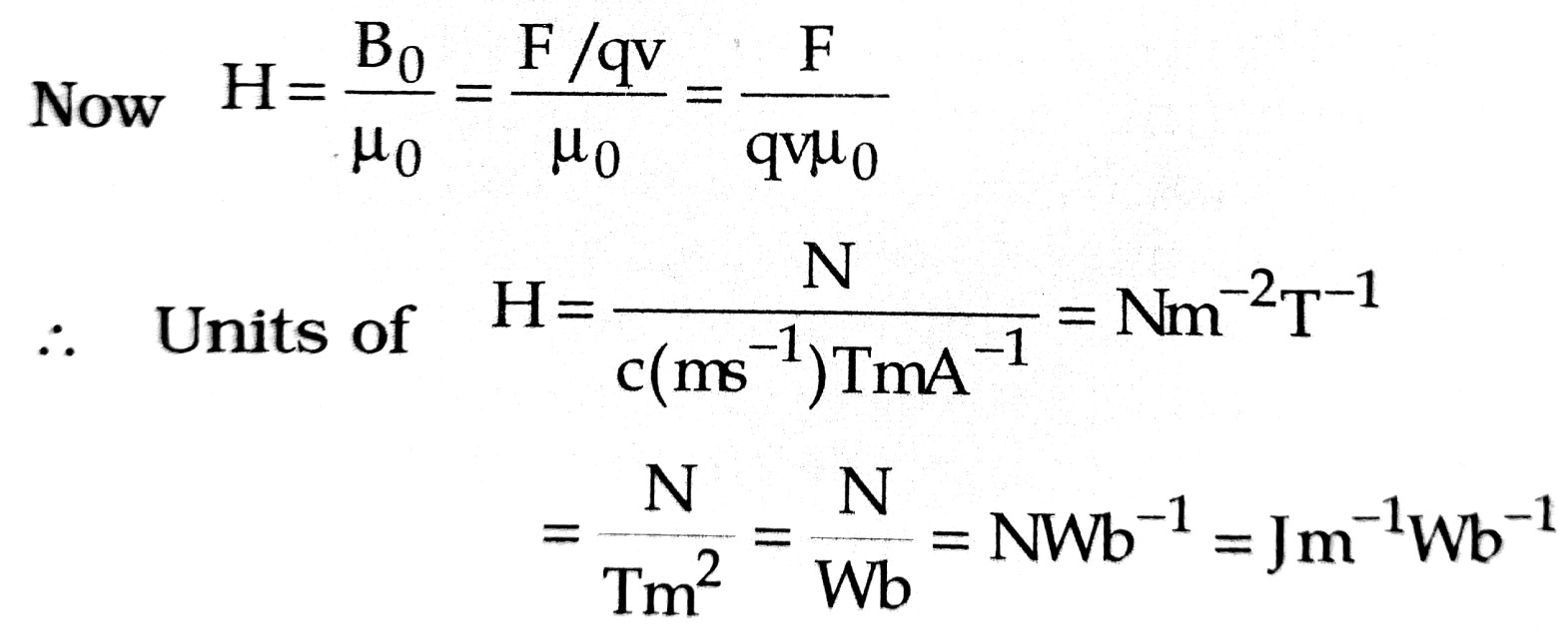Q. 17. Derive an expression for the potential energy of a magnetic dipole placed in a uniform magnetic field at an angle θ with it.

Ans ⇒
The torque acting on a magnetic dipole of a moment M held at an angle θ with the
direction of a uniform magnetic field B is given by
τ = MB sin θ
This torque tends to align the magnetic dipole in the direction of the field. Work has to be done in rotating the dipole against the action of the torque. This work done is stored as potential energy of the dipole.
The small amount of work done in rotating the dipole through angle do is given by
δW = τdθ = MB sin θ. dθ
Total work done in rotating the dipole from angle θ1 to θ2 is given by :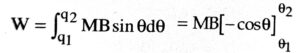W=-MB[cosθ2 – cosθ1]
When dipole is rotated from θ1 = 90° to θ2 = θ,
then         W = -MB[cosθ – cos90°] = -MB cosθ.
∴   Potential energy of dipole V(=W) = -MB cosθ
→  →
In vector rotation V = -M. B.

## Class 12th physics Subjective question in English

 S.N Physics Short Type Question English Medium 1. ELECTRIC CHARGES AND FIELDS 2. LECTROSTATIC POTENTIAL AND CAPACITANCE 3. CURRENT ELECTRICITY 4. MOVING CHARGES AND MAGNETISM 5. MAGNETISM AND MATTER 6. ELECTROMAGNETIC INDUCTION 7. ALTERNATING CURRENT 8. ELECTROMAGNETIC WAVES 9. RAY OPTICS AND OPTICAL INSTRUMENTS 10. WAVE OPTICS 11. DUAL NATURE OF MATTER AND RADIATION 12. ATOMS 13. NUCLEI 14. SEMI CONDUCTOR ELECTRONICS 15. COMMUNICATION SYSTEMS